# Plotting graphs of the functions

Click on the «draw» button to display the graph of the function

### How to use

With the help of this program at Cubens you can build the graph of the function online.

• decimal fractions you need to divide via point
• in some cases it is possible not to write the multiplication signs
• you can build a variety of graphs of functions simultaneously
• you can customize the names and intervals of the axes
• graph can be printed
• you can obtain a link to the graph to share it with others
• when the cursor is on the graph you can move it and increase or decrease the scale

### Modes

Currently, the program provides four modes:

• Simple — in this mode, you can draw graphs of functions defined by the equation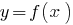• Parametric — allows you to plot curves given parametrically, i.e., in the form of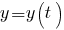• Polar coordinates — allows you to plot curves given in polar coordinates, the equation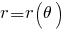, where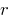is the radial coordinate and the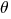is the polar coordinate
• Points — this mode is intended for plotting functions specifying the coordinates of their points

### The graph of the function

Dependency variableof variable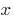is called a function if to each value ofcorresponds to a single value of.

The function is denoted by a single letter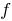,or.

The domain of the function is all values that can take an argument (variable).

The value of the function is all values that a function can take (variable) for allfrom the field definition of the function.

The function can be set using the table, graph, or formula. The formula specifies a rule that each value of argumentis assigned the value of the function.

The graph of the functionis the set of all points in the coordinate plane with coordinates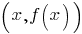, where the first coordinate ofruns all scope of options of, and the second coordinate is the corresponding value functionat the point.

### Operators

Value Description
`+` addition
`-` subtraction
`*` multiplication
`/` division
`^n` exponentiation n
`^(1/n)` root of n-th degree
`()` brackets

### Functions

Value Description
`abs(x)` module x
`sqrt(x)` square root of x
`nthRoot(x,n)` root of n-th degree of x
`pow(x,n)` construction of x to power n
`exp(x)` exponent of x (Euler's number to power of x)
`log2(x)` base 2 logarithm of x
`log10(x)` base 10 logarithm of x
`ln(x)` natural logarithm of x
`min(...)` minimum value from set of numbers
`max(...)` maximum value from set of numbers

### Trigonometric functions

Value Description
`sin(x)` sine of x
`cos(x)` cosine of x
`tan(x)` tangent of x
`1/tan(x)` cotangent of x
`asin(x)` arc sine of x
`acos(x)` arc cosine of x
`atan(x)` arc tangent of x
`PI_HALF-atan(x)` arc cotangent of x
`sinh(x)` hyperbolic sine of x
`cosh(x)` hyperbolic cosine of x
`tanh(x)` hyperbolic tangent of x
`1/tanh(x)` hyperbolic cotangent of x

### Constants

Value Description
`PI` number Pi (3,14159...)
`PI_HALF` number Pi divided by 2
`PI_TWICE` number Pi multiplied by 2
`ONE` one
`ZERO` zero
Share with friends: